Скачать презентацию Exam III Physics 101 Lecture 17 Fluids Exam

221c7d96b51ec410476dfedb04cc111f.ppt

• Количество слайдов: 15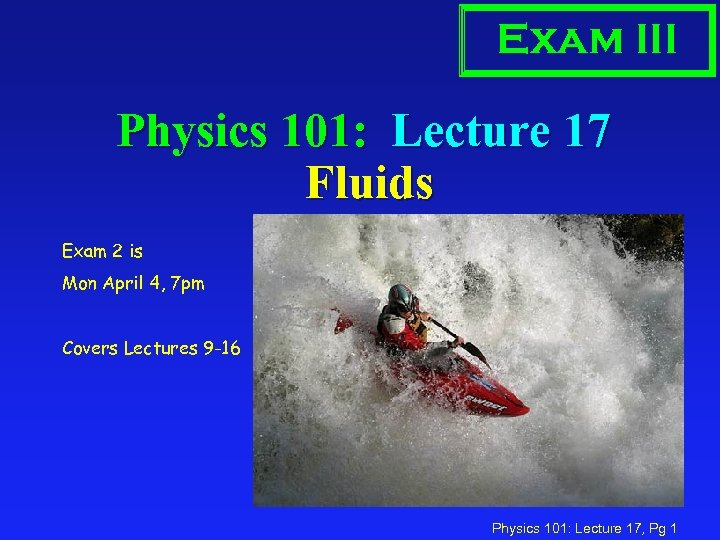Exam III Physics 101: Lecture 17 Fluids Exam 2 is Mon April 4, 7 pm Covers Lectures 9 -16 Physics 101: Lecture 17, Pg 1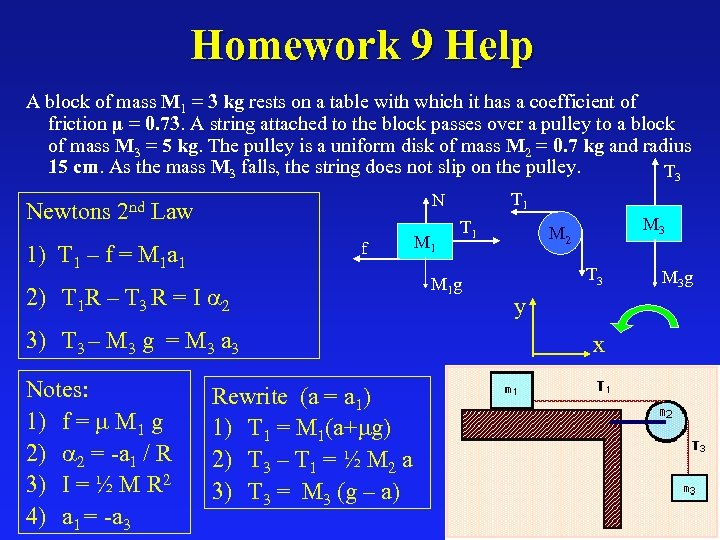Homework 9 Help A block of mass M 1 = 3 kg rests on a table with which it has a coefficient of friction µ = 0. 73. A string attached to the block passes over a pulley to a block of mass M 3 = 5 kg. The pulley is a uniform disk of mass M 2 = 0. 7 kg and radius 15 cm. As the mass M 3 falls, the string does not slip on the pulley. T 3 f 1) T 1 – f = M 1 a 1 2) T 1 R – T 3 R = I a 2 3) T 3 – M 3 g = M 3 a 3 Notes: 1) f = m M 1 g 2) a 2 = -a 1 / R 3) I = ½ M R 2 4) a 1 = -a 3 T 1 N Newtons 2 nd Law M 1 T 1 M 1 g M 3 M 2 T 3 M 3 g y x Rewrite (a = a 1) 1) T 1 = M 1(a+mg) 2) T 3 – T 1 = ½ M 2 a 3) T 3 = M 3 (g – a) Physics 101: Lecture 17, Pg 2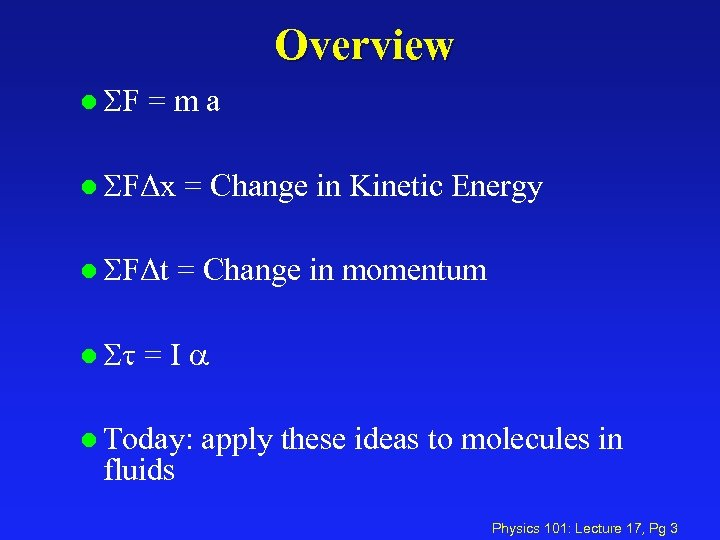Overview l SF =ma l SFΔx = Change in Kinetic Energy l SFΔt = Change in momentum l St =Ia l Today: fluids apply these ideas to molecules in Physics 101: Lecture 17, Pg 3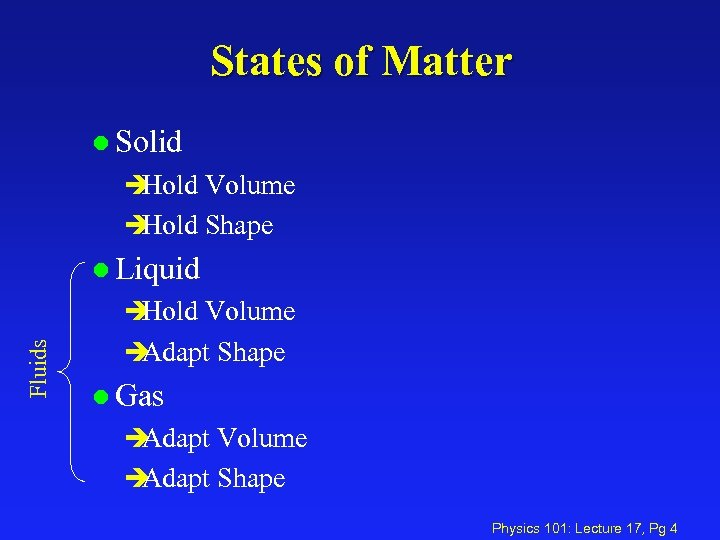States of Matter l Solid è Hold Volume è Hold Shape l Liquid Fluids è Hold Volume è Adapt Shape l Gas è Adapt Volume è Adapt Shape Physics 101: Lecture 17, Pg 4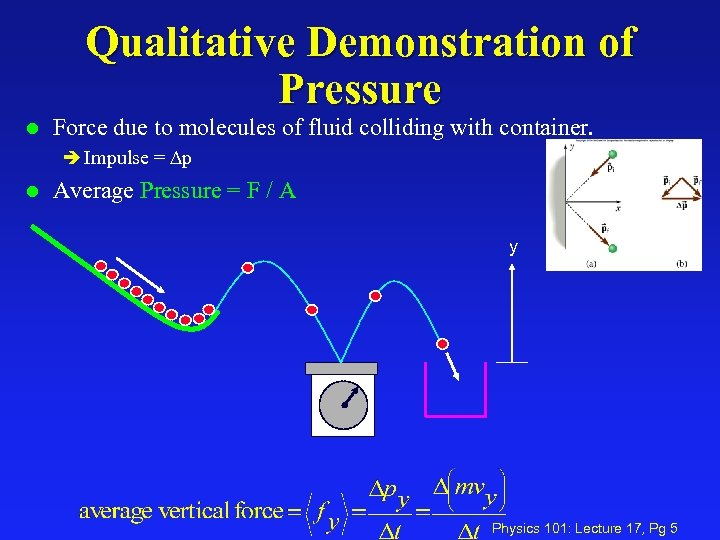Qualitative Demonstration of Pressure l Force due to molecules of fluid colliding with container. è Impulse = Dp l Average Pressure = F / A y Physics 101: Lecture 17, Pg 5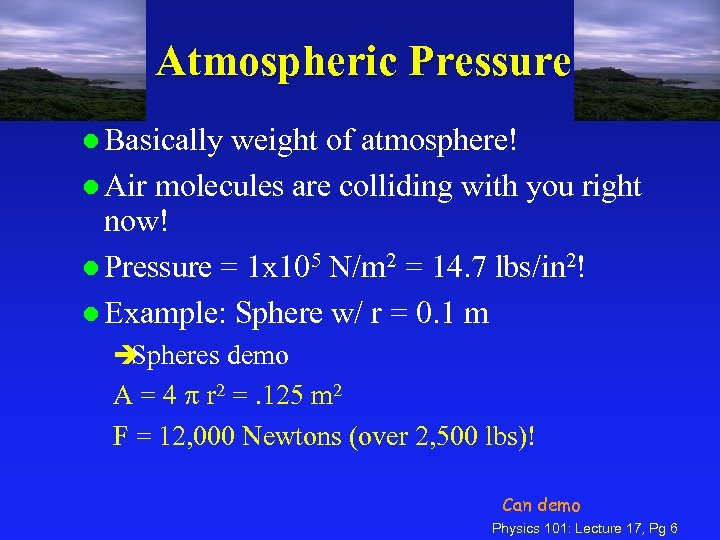Atmospheric Pressure l Basically weight of atmosphere! l Air molecules are colliding with you right now! l Pressure = 1 x 105 N/m 2 = 14. 7 lbs/in 2! l Example: Sphere w/ r = 0. 1 m è Spheres demo A = 4 p r 2 =. 125 m 2 F = 12, 000 Newtons (over 2, 500 lbs)! Can demo Physics 101: Lecture 17, Pg 6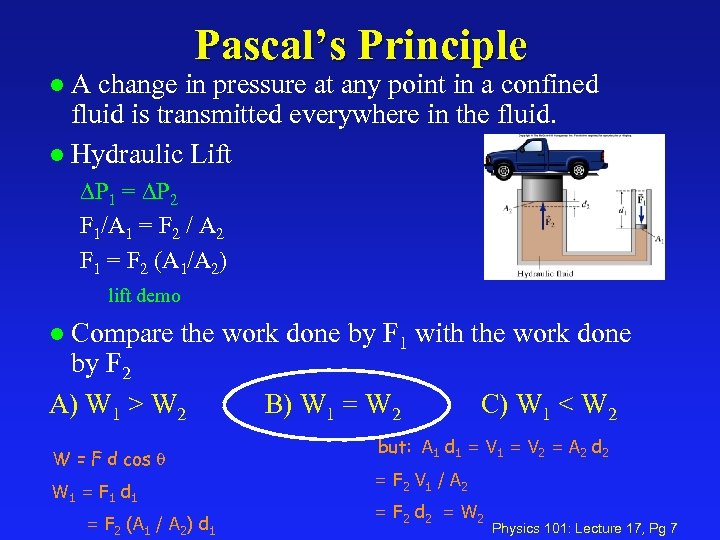Pascal’s Principle A change in pressure at any point in a confined fluid is transmitted everywhere in the fluid. l Hydraulic Lift l DP 1 = DP 2 F 1/A 1 = F 2 / A 2 F 1 = F 2 (A 1/A 2) lift demo Compare the work done by F 1 with the work done by F 2 A) W 1 > W 2 B) W 1 = W 2 C) W 1 < W 2 l W = F d cos q W 1 = F 1 d 1 = F 2 (A 1 / A 2) d 1 but: A 1 d 1 = V 2 = A 2 d 2 = F 2 V 1 / A 2 = F 2 d 2 = W 2 Physics 101: Lecture 17, Pg 7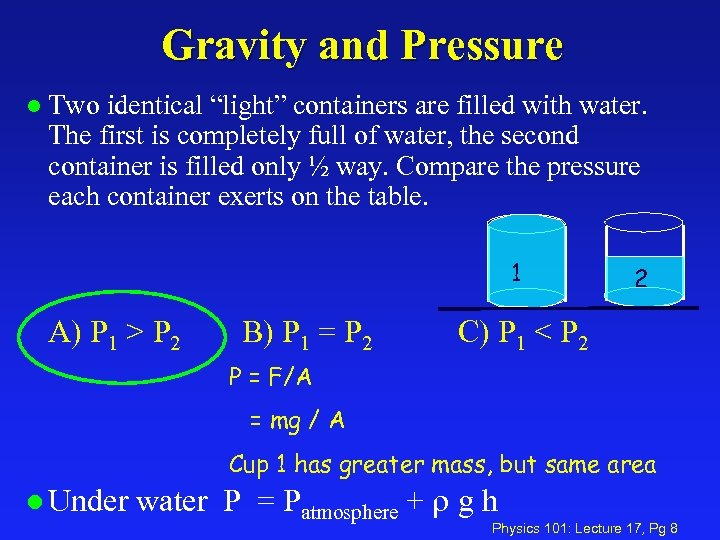Gravity and Pressure l Two identical “light” containers are filled with water. The first is completely full of water, the second container is filled only ½ way. Compare the pressure each container exerts on the table. 1 A) P 1 > P 2 B) P 1 = P 2 2 C) P 1 < P 2 P = F/A = mg / A Cup 1 has greater mass, but same area l Under water P = Patmosphere + g h Physics 101: Lecture 17, Pg 8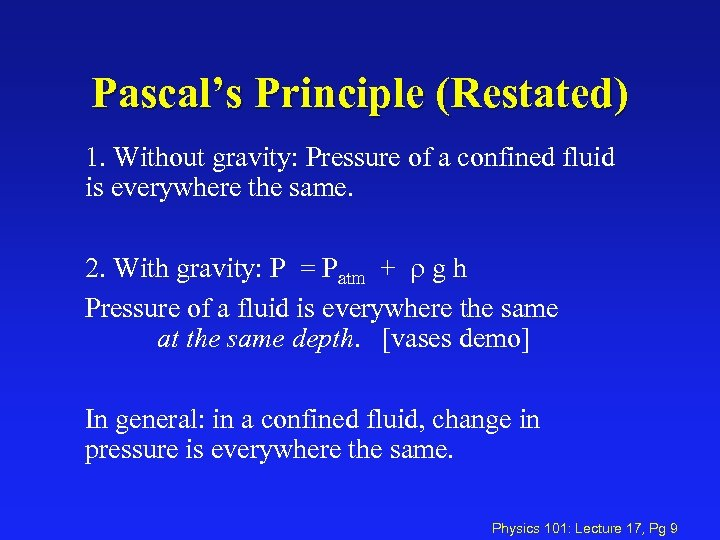Pascal’s Principle (Restated) 1. Without gravity: Pressure of a confined fluid is everywhere the same. 2. With gravity: P = Patm + g h Pressure of a fluid is everywhere the same at the same depth. [vases demo] In general: in a confined fluid, change in pressure is everywhere the same. Physics 101: Lecture 17, Pg 9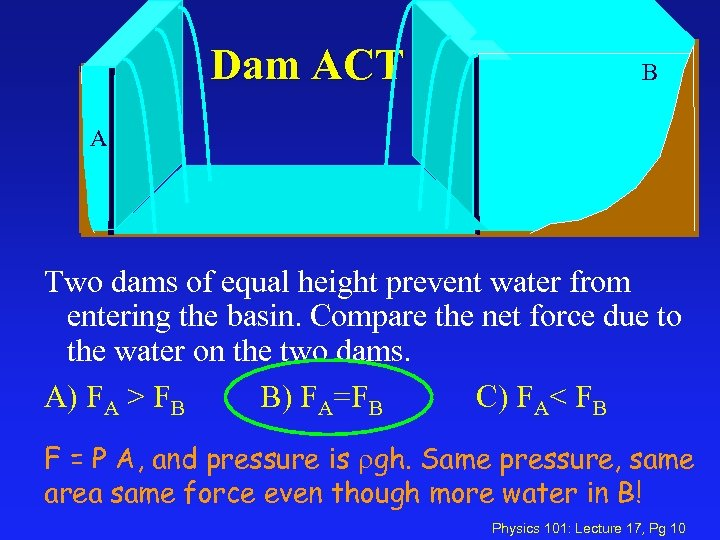Dam ACT B A A Two dams of equal height prevent water from entering the basin. Compare the net force due to the water on the two dams. A) FA > FB B) FA=FB C) FA< FB F = P A, and pressure is gh. Same pressure, same area same force even though more water in B! Physics 101: Lecture 17, Pg 10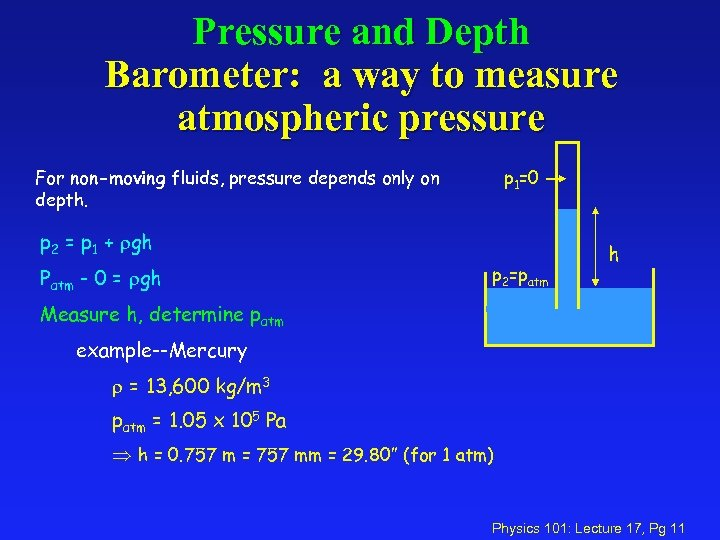Pressure and Depth Barometer: a way to measure atmospheric pressure For non-moving fluids, pressure depends only on depth. p 1=0 p 2 = p 1 + gh Patm - 0 = gh p 2=patm h Measure h, determine patm example--Mercury = 13, 600 kg/m 3 patm = 1. 05 x 105 Pa h = 0. 757 m = 757 mm = 29. 80” (for 1 atm) Physics 101: Lecture 17, Pg 11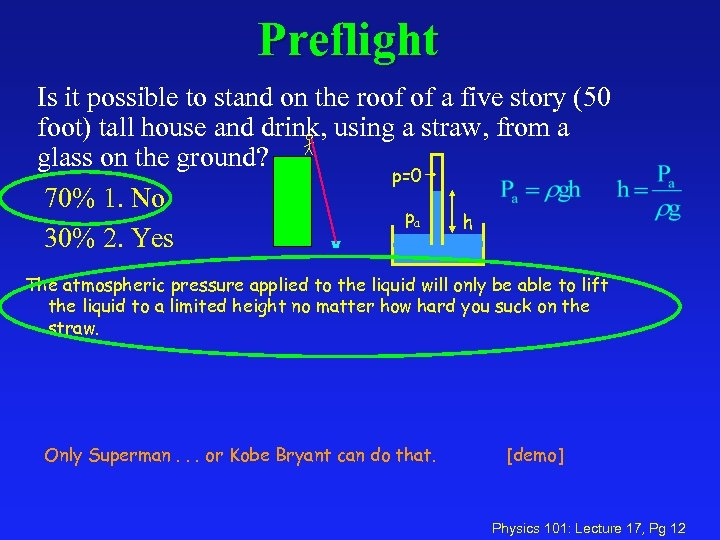Preflight Is it possible to stand on the roof of a five story (50 foot) tall house and drink, using a straw, from a glass on the ground? p=0 70% 1. No pa h 30% 2. Yes The atmospheric pressure applied to the liquid will only be able to lift the liquid to a limited height no matter how hard you suck on the straw. Only Superman. . . or Kobe Bryant can do that. [demo] Physics 101: Lecture 17, Pg 12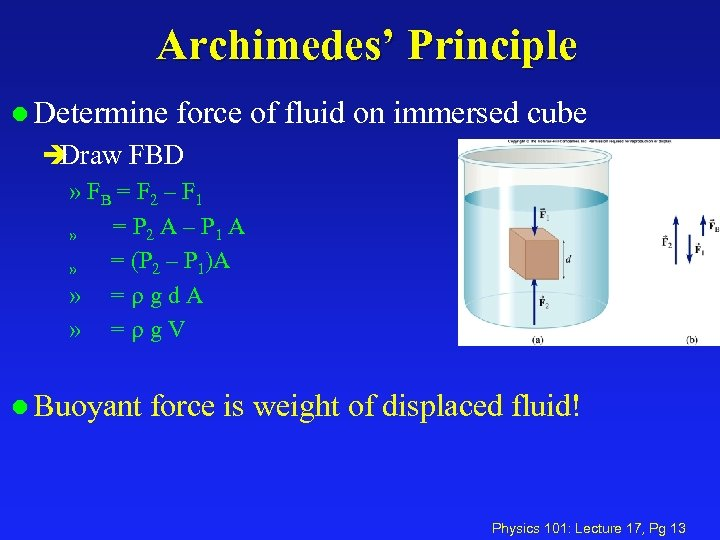Archimedes’ Principle l Determine force of fluid on immersed cube è Draw FBD » FB = F 2 – F 1 » » = P 2 A – P 1 A = (P 2 – P 1)A = gd. A = g. V l Buoyant force is weight of displaced fluid! Physics 101: Lecture 17, Pg 13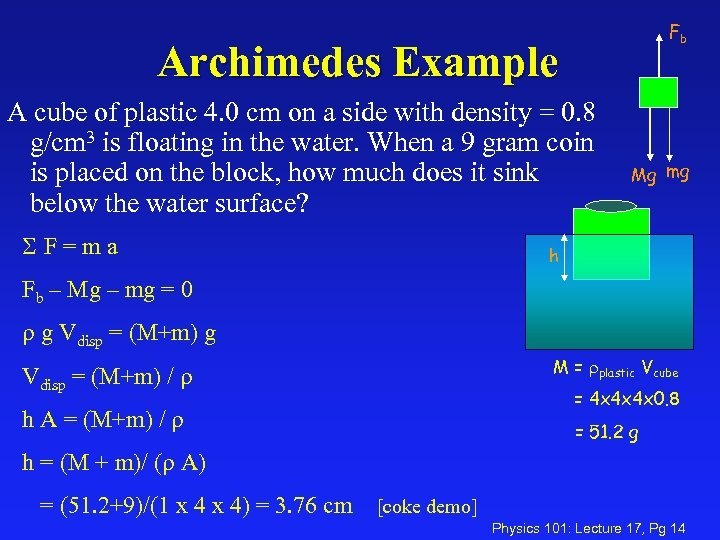Fb Archimedes Example A cube of plastic 4. 0 cm on a side with density = 0. 8 g/cm 3 is floating in the water. When a 9 gram coin is placed on the block, how much does it sink below the water surface? SF=ma Mg mg h Fb – Mg – mg = 0 g Vdisp = (M+m) g M = plastic Vcube Vdisp = (M+m) / = 4 x 4 x 4 x 0. 8 h A = (M+m) / = 51. 2 g h = (M + m)/ ( A) = (51. 2+9)/(1 x 4) = 3. 76 cm [coke demo] Physics 101: Lecture 17, Pg 14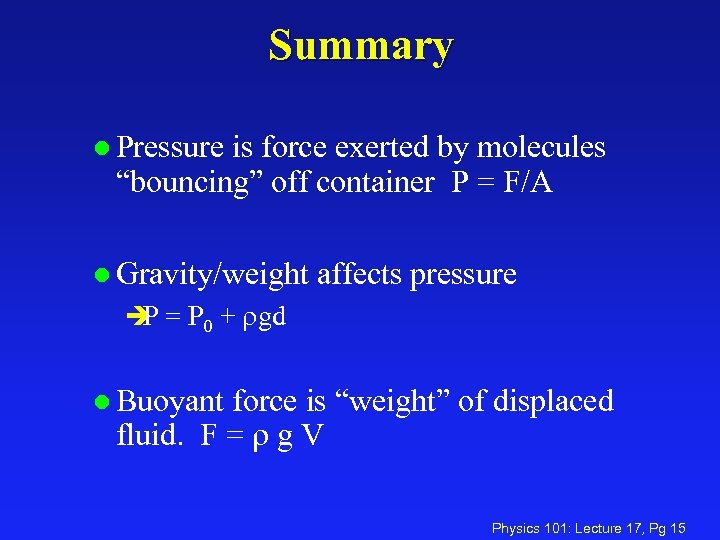Summary l Pressure is force exerted by molecules “bouncing” off container P = F/A l Gravity/weight affects pressure è = P 0 + gd P l Buoyant force is “weight” of displaced fluid. F = g V Physics 101: Lecture 17, Pg 15